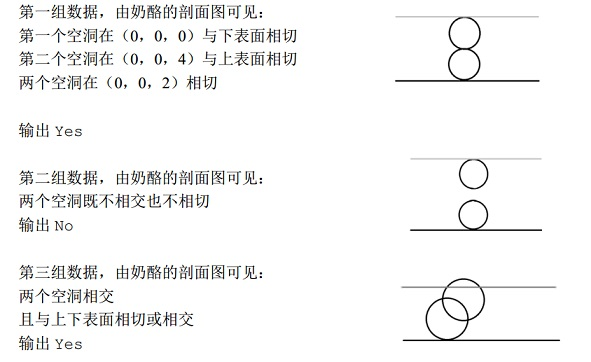【题目描述】

dist(P1,P2)=sqrt((x1−x2)^2+(y1−y2)^2+(z1−z2)^2)
【输入】

【输出】

【输入样例】
3
2 4 1
0 0 1
0 0 3
2 5 1
0 0 1
0 0 4
2 5 2
0 0 2
2 0 4
【输出样例】
Yes
No
Yes
【提示】#include <iostream>
#include <queue>
using namespace std;

long long n, h, R, Rs;

struct point
{
long long x, y, z;
inline long long dist(const point &n) //算出平方距离
{
return (this->x - n.x)  (this->x - n.x) + (this->y - n.y)  (this->y - n.y) + (this->z - n.z) * (this->z - n.z);
}
};
struct ball
{
point Point;

bool ved;

inline bool operator|(const ball &n) //连通
{
long long dis = this->Point.dist(n.Point);
if (dis > Rs)
return false;
return true;
}

inline bool istop()
{
if (this->Point.z + R >= h)
return true;
return false;
}

inline bool isstart()
{
if (this->Point.z <= R)
return true;
return false;
}

} Ball;

bool bfs(int start)
{
queue<int> Q;
Q.push(start);
while (!Q.empty())
{
int t = Q.front();
Q.pop();
if (Ball[t].ved)
continue;
Ball[t].ved = true;

if (Ball[t].istop())
return true;

for (int i = 0; i < n; i++)
if (!Ball[i].ved && Ball[i] | Ball[t])
Q.push(i);
}
return false;
}

int main()
{
int M;
cin >> M;
while (M--)
{
cin >> n >> h >> R;
Rs = 4  R  R;
for (int i = 0; i < n; i++)
{
cin >> Ball[i].Point.x >> Ball[i].Point.y >> Ball[i].Point.z;
Ball[i].ved = false;
}
for (int i = 0; i < n; i++)
{
if (Ball[i].isstart())
if (bfs(i))
{
cout << "Yes" << endl;
goto End;
}
}
cout << "No" << endl;
End:
;
}

return 0;
}

Last modification：February 7th, 2021 at 02:08 pm